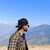# Calculators Created by Himanshu SharmaNational Institute of Technology, Hamirpur (NITH), Himachal Pradesh
95
Formulas Created
0
Formulas Verified
8
Across Categories

## List of Calculators by Himanshu Sharma

Following is a combined list of all the calculators that have been created and verified by Himanshu Sharma. Himanshu Sharma has created 95 and verified 0 calculators across 8 different categories till date.
Created Aerodynamic force
Created Ambient air density given dynamic pressure
Created Ambient air density given gas constant
Created Ambient air density given mach number
Created Aspect ration in lateral plane
Created Flat plate area
Created Flight speed given dynamic pressure
Created Form factor given flat plate area
Created Gas constant given dynamic pressure
Created Gross weight given drag
Created Induced drag
Created Lateral plane span given aspect ratio
Created Lateral plane span given induced drag
Created Mach number given gas constant
Created Normal pressure given dynamic pressure
Created Skin friction coefficient given flat plate area
Created Specific heat of air given gas constant new
Created Specific heat of air given normal pressure
Created Temperature given gas constant
Created Wetted area given aspect ratio
Created Wetted area given flat plate area
1 More Aerodynamic Drag and its Reduction Calculators
Created Fuel load from unity equation
Created Landing weight given take off weight and mission fuel weight
Created Landing weight given zero fuel weight and reserve fuel weight
Created Maximum take off weight
Created Mission fuel
Created Mission fuel given take off, reserve and zero fuel weight
Created Operating empty weight from unity equation
Created Payload weight from Unity equation
Created Range increment of aircraft
Created Reserve fuel
Created Take off weight from Unity equation
Created Take off weight given reserve, mission and zero fuel weight
Created Zero fuel weight given mission fuel, reserve and take off weight
Created Zero fuel weight given reserve and landing weight
6 More Conceptual Design Calculators
Drag (7)
Created Coefficient of drag given drag
Created Drag
Created Drag coefficient given lift coefficient
Created Drag coefficient new
Created Drag given aerodynamic force
Created Drag given drag coefficient
Created Drag given lift coefficient
Created Dynamic pressure aircraft
Created Dynamic pressure given drag coefficient
Created Dynamic pressure given gas constant
Created Dynamic pressure given induced drag
Created Dynamic pressure given lift coefficient
Created Dynamic pressure given mach number
Created Dynamic pressure given normal pressure
Lift (7)
Created Coefficient of lift given drag
Created Lift coefficient given drag coefficient
Created Lift coefficient new
Created Lift given aerodynamic force
Created Lift given drag coefficient
Created Lift given induced drag
Created Lift given lift coefficient
Created Mass flow rate cool secondary given turbofan thrust
Created Mass flow rate given ideal thrust
Created Mass flow rate given ram drag and flight speed
Created Mass flow rate given thrust
Created Mass flow rate hot primary engine
Created Mass flow rate hot primary given turbofan thrust
Created Mass flow rate of ambient air
Created Mass flow rate of cool secondary engine
Created Mass flow rate of turbojet thrust
Created Area of nozzle exit of turbojet
Created Design range given range increment
Created Expansion velocity cool secondary given turbofan thrust
Created Expansion velocity given thrust
Created Expansion velocity hot primary given turbofan thrust
Created Flight speed given ideal thrust
Created Flight speed given momentum of ambient air
Created Flight speed given ram drag and mass flow rate
Created Flight speed given thrust
Created Gross thrust of turbojet given net thrust and ram drag
Created Harmonic range given range increment
Created Ideal thrust of turbojet
Created Momentum of ambient air
Created Net thrust of turbojet given gross thrust and ram drag
Created Ram drag
Created Ram drag of turbojet given gross thrust and net thrust
Created Thrust exerted by engine
Created Total mass flow rate through turbofan engine
Created Turbofan thrust
Created Turbojet gross thrust
Created Velocity after expansion given ideal thrust
Created Velocity of turbojet
Created Gross weight
Created Operating empty weight
Created Operating empty weight considering zero fuel weight
Created Zero fuel weight
3 More Weight Terminologies of Aircraft Design CalculatorsLet Others Know